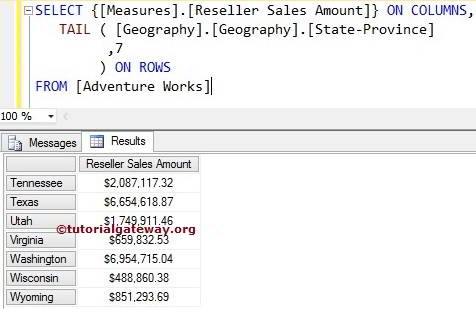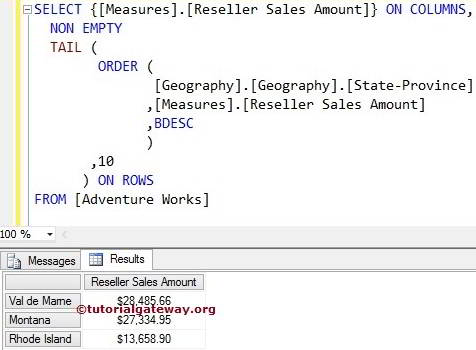# MDX Tail Function

The MDX Tail Function used to select the required number of records from the bottom of the source data. For example, If you want to find the Bottom 10 performing products. Or, find the Bottom 10 regions with Lowest sales or to calculate Lowest Salary, use this MDX Tail function along with Order Function.

## MDX Tail Function Syntax

The MDX Tail Function allows only two arguments. The basic syntax of this TAIL function in Multidimensional Expression is as shown below:

`TAIL (Set_Expression, Count)`
• Set_Expression: Any Multidimensional Expression or attributes on which you want to check.
• Count: Number of records you want to retrieve.

How to write MDX TAIL Function to extract Bottom 7 States from Geography dimension in the Adventure Works Cube whose Reseller Sales amount is lower than others with example?. For this MDX TAIL example, we are going to use the below-shown data.

## MDX Tail Function Example

In this example, we will show how to write the Tail function. The following query will return the Last seven records from the above source in the order they appear above.

```SELECT {[Measures].[Reseller Sales Amount]} ON COLUMNS,
TAIL (
[Geography].[Geography].[State-Province]
,7
) ON ROWS

In the above MDX Query, We selected the [Reseller Sales Amount] Measure on the Columns and [State Province] attribute present in the geography Dimension on Rows. Next, the Tail function will select the Bottom 7 records irrespective of their measured values.No one will accept the above result as the Least performing states around the world. They were just the Bottom 7 records.

## Using Order Function along with MDX Tail Function

In this example, we show you, What happens when we add the MDX Order Function.

```SELECT {[Measures].[Reseller Sales Amount]} ON COLUMNS,
ORDER (
,[Measures].[Reseller Sales Amount], DESC
) ON ROWS

First, the MDX Tail function will select the Last 7 records present in the source data using the below statement

` TAIL ([Geography].[Geography].[State-Province], 7)`

Next, Order function outside the Tail function will sort the already picked data in the descending order using their Reseller Sales Amount.

``` ORDER (
TAIL ([Geography].[Geography].[State-Province], 7)
,[Measures].[Reseller Sales Amount], DESC
) ON ROWS```

It means we are getting the Last seven records from the source data in Descending order

Let us change the above MDX code:

```SELECT {[Measures].[Reseller Sales Amount]} ON COLUMNS,
TAIL (
ORDER (
[Geography].[Geography].[State-Province]
,[Measures].[Reseller Sales Amount]
,BDESC
)
,7
) ON ROWS

First, the Order function will sort the State Province data in the Descending order using their Reseller Sales Amount. We used BDESC to break the hierarchy while sorting the data.

```ORDER (
[Geography].[Geography].[State-Province]
,[Measures].[Reseller Sales Amount]
,BDESC
)```

Next, MDX Tail function will pick the Last 7 records from the already sorted data.

```TAIL (
ORDER (
[Geography].[Geography].[State-Province]
,[Measures].[Reseller Sales Amount]
,BDESC
)
,7
) ON ROWS
```

Now, We got our Bottom 7 records or Least performing states.

NOTE: Please use the appropriate Measure as the second argument in the ORDER function. Otherwise, You will end up with the wrong results.

## Using NON EMPTY in MDX Tail Function

In this example, we are going to use the Non Empty keyword to remove the NULL values.

```SELECT {[Measures].[Reseller Sales Amount]} ON COLUMNS,
NON EMPTY
TAIL (
ORDER (
[Geography].[Geography].[State-Province]
,[Measures].[Reseller Sales Amount]
,BDESC
)
,7
) ON ROWS